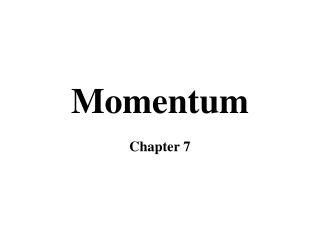DownloadDownload PresentationMomentum

# Momentum

Download Presentation## Momentum

- - - - - - - - - - - - - - - - - - - - - - - - - - - E N D - - - - - - - - - - - - - - - - - - - - - - - - - - -
##### Presentation Transcript

1. Momentum Chapter 7

2. Momentum “Inertia in Motion” mass X velocity mv

3. Momentum mass X velocity mv If traveling at the same speed, which has more momentum?

4. Momentum What if they had the same momentum? mv = mv

5. What is the momentum of a 0.50 kg kitten running with a velocity of 1.00 m/s to the left?

6. What is the momentum of a 0.50 kg kitten running with a velocity of 1.00 m/s to the left? Given: Substitute: m = 0.50 kg p = (0.50 kg)(1.00 m/s) v = 1.00 m/s Unknown: Solve: p (momentum) p = 0.5 kgm/s Equation: p = mv

7. Jenny has a mass of 35.6 kg and her skateboard has a mass of 1.3 kg. What is the momentum of Jenny and her skateboard together if they are going 9.50 m/s?

8. Jenny has a mass of 35.6 kg and her skateboard has a mass of 1.3 kg. What is the momentum of Jenny and her skateboard together if they are going 9.50 m/s? Given: Equation: m = 36.9 kg p=mv v = 9.50 m/s Substitute: Unknown: p = (36.9 kg)(9.50 m/s) p (momentum) Solve: p = 350.55 kgm/s

9. How do we change momentum? • Change in mass • example: items falling out of a truck • Change in velocity

10. What is the term for change in velocity? • Acceleration And what causes acceleration? FORCES!

11. Impulse • Any change in momentum, but usually occurs as a change in speed What besides force would cause a change in momentum? TIME! Ft

12. Impulse v = 20 m/s v = 20 m/s Which would you rather hit?

13. Impulse Ft And you look like:

14. Impulse Ft And you look like:

15. Impulse

16. While being thrown, a net force of 132 N acts on a baseball (mass = 140 g) for a period of 4.5 X 10-2 sec. What is the magnitude of the change in momentum of the ball?

17. When the batter hits the ball, a net force of 1320 N, opposite to the direction of the ball’s initial motion, acts on the ball for 9.0 X 10-3 s during the hit. What is the change in momentum of the ball? What force does the ball exert on the bat in the question above?

18. A 1.00 kg ball traveling at a velocity of 3.00 m/s west strikes a wall and rebounds with a velocity of 3.00 m/s east. The ball contracts the wall for 0.01 s. • What is the ball’s momentum before it hits the wall?

19. A 1.00 kg ball traveling at a velocity of 3.00 m/s west strikes a wall and rebounds with a velocity of 3.00 m/s east. The ball contracts the wall for 0.01 s. • What is the ball’s momentum before it hits the wall? -3 kgm/s • What is the ball’s momentum after it hits the wall?

20. A 1.00 kg ball traveling at a velocity of 3.00 m/s west strikes a wall and rebounds with a velocity of 3.00 m/s east. The ball contracts the wall for 0.01 s. • What is the ball’s momentum before it hits the wall? -3 kgm/s • What is the ball’s momentum after it hits the wall? 3 kgm/s • What is the ball’s change in momentum?

21. A 1.00 kg ball traveling at a velocity of 3.00 m/s west strikes a wall and rebounds with a velocity of 3.00 m/s east. The ball contracts the wall for 0.01 s. • What is the ball’s momentum before it hits the wall? -3 kgm/s • What is the ball’s momentum after it hits the wall? 3 kgm/s • What is the ball’s change in momentum? 6 Ns • What force must the wall exert on the ball in order to change its momentum?

22. Bouncing Requires more impulse than stopping.

23. Bouncing The flat paddle just stops the water.

24. Bouncing The curved paddle causes the water to make a U-turn or bounce.

25. Impulse Question On a sheet of paper, answer the following question, using what was discussed yesterday about impulse and momentum. Which would you rather hit and why? or

26. Conservation of Momentum -mv = mv Before firing:After firing: momentum = 0 momentum = 0 0 + 0 = 0 mV + (-Mv) = 0

27. Conservation of Momentum • Law of Conservation of Momentum: In the absence of an external force, the momentum of a system remains unchanged. • If no external forces are applied to the system, momentum is conserved.

28. Elastic collisions Momentum is conserved The total momentum for the system does not change Energy is also conserved Collisions

29. Elastic collisions Basically, if A and B have the same masses, they simply swap velocities after the collision. Collisions

30. Inelastic collisions Momentum is still conserved, but energy is lost due to an outside force (like friction) Usually occurs when there is a change in mass or when the two masses combine to form one object. Collisions

31. Collisions

32. If the objects have the same mass. With elastic collisions, starting and ending velocities are equal. With inelastic collisions, starting velocities are reduced Collisions